# Concatenate multiIndex into single index in Pandas Series

In this article, we will see how to concatenate multi-index to a single index in Pandas Series. Multi-index refers to having more than one index with the same name.

Create a sample series:

## Python3

 `# importing pandas module` `import` `pandas as pd` `import` `numpy as np`   `# Creating series data for address details` `index_values ``=` `pd.Series([(``'sravan'``, ``'address1'``),` `                          ``(``'sravan'``, ``'address2'``),` `                          ``(``'sudheer'``, ``'address1'``),` `                          ``(``'sudheer'``, ``'address2'``)])`   `# assigning values with integers` `data ``=` `pd.Series(np.arange(``1``, ``5``),` `                 ``index``=``index_values)`   `# display data` `print``(data)`

Output: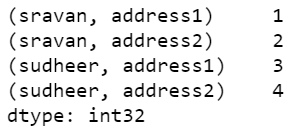Joining two or more data is known as concatenation. Here we are going to concatenate the index using map function.

Syntax:

map(fun, iter)

• fun: function
• iter: iterations.

Below are various examples that depict how to concatenate multi-index into a single index in Series:

Example 1:

This code explains the joining of addresses into one based on multi-index.

## Python3

 `# importing pandas module` `import` `pandas as pd`   `# Creating series data for address details` `index_values ``=` `pd.Series([(``'sravan'``, ``'address1'``),` `                          ``(``'sravan'``, ``'address2'``),` `                          ``(``'sudheer'``, ``'address1'``),` `                          ``(``'sudheer'``, ``'address2'``)])`   `# assigning values with integers` `data ``=` `pd.Series(np.arange(``1``, ``5``), index``=``index_values)`   `# display data` `print``(data)`   `# mapping with data using '_' symbol with join` `data1 ``=` `data.index.``map``(``'_'``.join)`   `print``(data1)`

Output: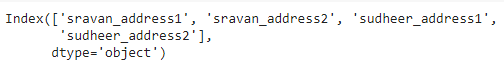Example 2:

This code is an example for all the given same name, but different values passed in a tuple.

## Python3

 `# importing pandas module` `import` `pandas as pd`   `# importing numpy module` `import` `numpy as np`   `# Creating series data for address details with same name.` `index_values ``=` `pd.Series([(``'sravan'``, ``'address1'``), ` `                          ``(``'sravan'``, ``'address2'``), ` `                          ``(``'sravan'``, ``'address3'``), ` `                          ``(``'sravan'``, ``'address4'``)])`   `# assigning values with integers` `data ``=` `pd.Series(np.arange(``1``, ``5``), ` `                 ``index``=``index_values)`   `# display data` `print``(data)`   `# mapping with data using '_' symbol with join` `data1 ``=` `data.index.``map``(``'_'``.join)`   `print``(data1)`

Output: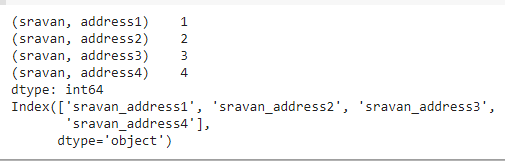Example 3:

This code gives a demo on multiple users given in nested list data structure.

## Python3

 `# importing pandas module` `import` `pandas as pd`   `# importing numpy module` `import` `numpy as np`   `# Creating series data for address details ` `# with same name with nested lists.` `index_values ``=` `pd.Series([[``'sravan'``, ``'address1'``], ` `                          ``[``'sravan'``, ``'address2'``], ` `                          ``[``'sravan'``, ``'address3'``], ` `                          ``[``'sravan'``, ``'address4'``], ` `                          ``[``'vani'``, ``'address5'``], ` `                          ``[``'vani'``, ``'address6'``], ` `                          ``[``'vani'``, ``'address7'``], ` `                          ``[``'vani'``, ``'address8'``]])`   `# assigning values with integers` `data ``=` `pd.Series(np.arange(``1``, ``9``), ` `                 ``index``=``index_values)`   `# display data` `print``(data)`   `# mapping with data using '_' symbol with join` `data1 ``=` `data.index.``map``(``'_'``.join)`   `print``(data1)`

Output: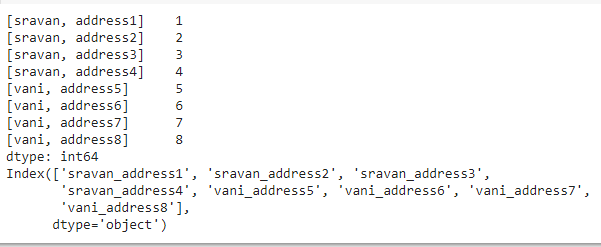Example 4:

This code explains the college data with respect to address passed in a nested list separated by ‘/’ operator.

## Python3

 `# importing pandas module` `import` `pandas as pd`   `# importing numpy module` `import` `numpy as np`   `# Creating series data for address details w.r.t ` `# college names  with same name with nested lists.` `index_values ``=` `pd.Series([[``'sravan'``, ``'address1'``, ``'vignan'``], ` `                          ``[``'sravan'``, ``'address2'``, ``'vignan'``], ` `                          ``[``'sravan'``, ``'address3'``, ``'vignan'``], ` `                          ``[``'sravan'``, ``'address4'``, ``'vignan'``], ` `                          ``[``'vani'``, ``'address5'``, ``'vignan lara'``], ` `                          ``[``'vani'``, ``'address6'``, ``'vignan lara'``], ` `                          ``[``'vani'``, ``'address7'``, ``'vignan lara'``], ` `                          ``[``'vani'``, ``'address8'``, ``'vignan lara'``]])`   `# assigning values with integers` `data ``=` `pd.Series(np.arange(``1``, ``9``), ` `                 ``index``=``index_values)`   `# display data` `print``(data)`   `# mapping with data using '/' symbol with join` `data1 ``=` `data.index.``map``(``'/'``.join)`   `print``(data1)`

Output: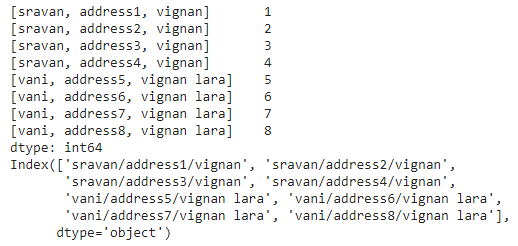Whether you're preparing for your first job interview or aiming to upskill in this ever-evolving tech landscape, GeeksforGeeks Courses are your key to success. We provide top-quality content at affordable prices, all geared towards accelerating your growth in a time-bound manner. Join the millions we've already empowered, and we're here to do the same for you. Don't miss out - check it out now!

Previous
Next